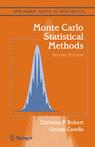## More typos in Monte Carlo Statistical MethodsFollowing my earlier post, I received further typos from Amir Alipour and Gholamhussein Gholami, a former student of mine now teaching in Iran, found in Monte Carlo Statistical Method, again concentrated in a limited number of pages:

“Page 37, Example 2.2,$X_n \in [0,1]$. Then$2X_n-1 \in [-1,1]$ should has the same behavior as a sequence of random numbers distributed according to the arcsine distribution not$X_n$ itself. Furthermore, in figure Fig.2.1, you have used a function$y_n=F(x_n)$ without explaining that what it is.
Page 52, Example 2.19, line 26. “Since the maximum …”. The power of$1-b$ should be$a-\alpha$, otherwise we will end by$b=a/(2a-\alpha)$. I didn’t get why it should attain its maxima!! while we are looking for the smallest bound.
Page 53, Example 2.20, line 10. The ratio should be$f/g_\alpha(z)=e^{\alpha(z-\underline{\mu})}e^{-z^2/2}$.
Page 53, Example 2.20, line 15. It seems Eq. 2.11 should be$\alpha^*=\frac{\underline{\mu}+\sqrt{\underline{\mu}^2+4}}{2}$.
Page 583, Negative Binomial distribution,$n+x+1$ should be$n+x-1$

and I have posted those new typos on the associated (Hall of Shame!) webpage. The last one is particularly embarrassing! Many thanks to those two Iranian readers!

As an aside, it seems the new edition of The Bayesian Choice, originally intended as a paperback, is now printed as a hardcover, which makes it a real deal at \$39 (or 30 euros)!!!

### One Response to “More typos in Monte Carlo Statistical Methods”

1.Eric Schmitt Says:

Dear Prof. Roberts,

This is probably old news to you so I apologize if you have been hearing about it for years. I am reading your so far great “The Bayesian Choice” and I noticed that it seems like figures in the Introduction are referred to incorrectly in the text. Namely, on page 4, “Consider the dataset depicted in Figure 1.1,” refers to Figure 1.1.1. Figure 1.1.1 is called Figure 1.1 in the following paragraph as well. More significantly, Figure 1.1.2 is referred to as Figure 1.1.6 in Example 1.1.6.

I know this kind of error mining is annoying, especially something like this, but I thought I would mention it since I am reading through a new copy and maybe that means it hasn’t been corrected yet (I got the great deal hardcover).

Sorry again for this,

Eric Schmitt

This site uses Akismet to reduce spam. Learn how your comment data is processed.